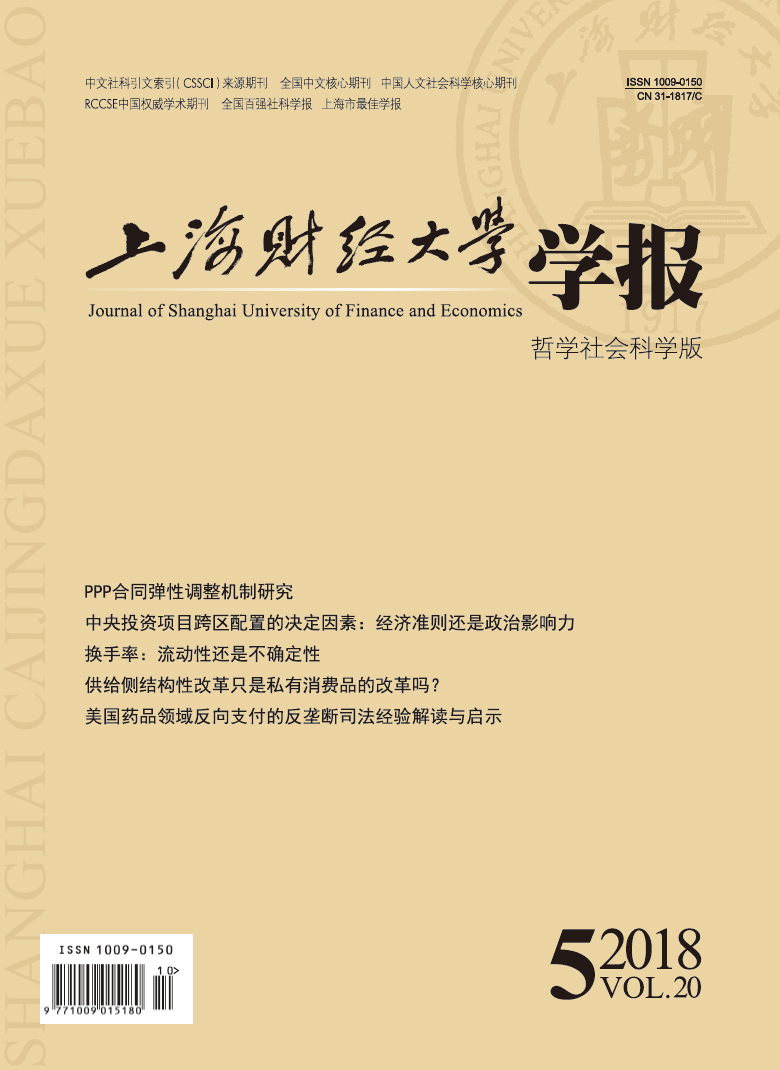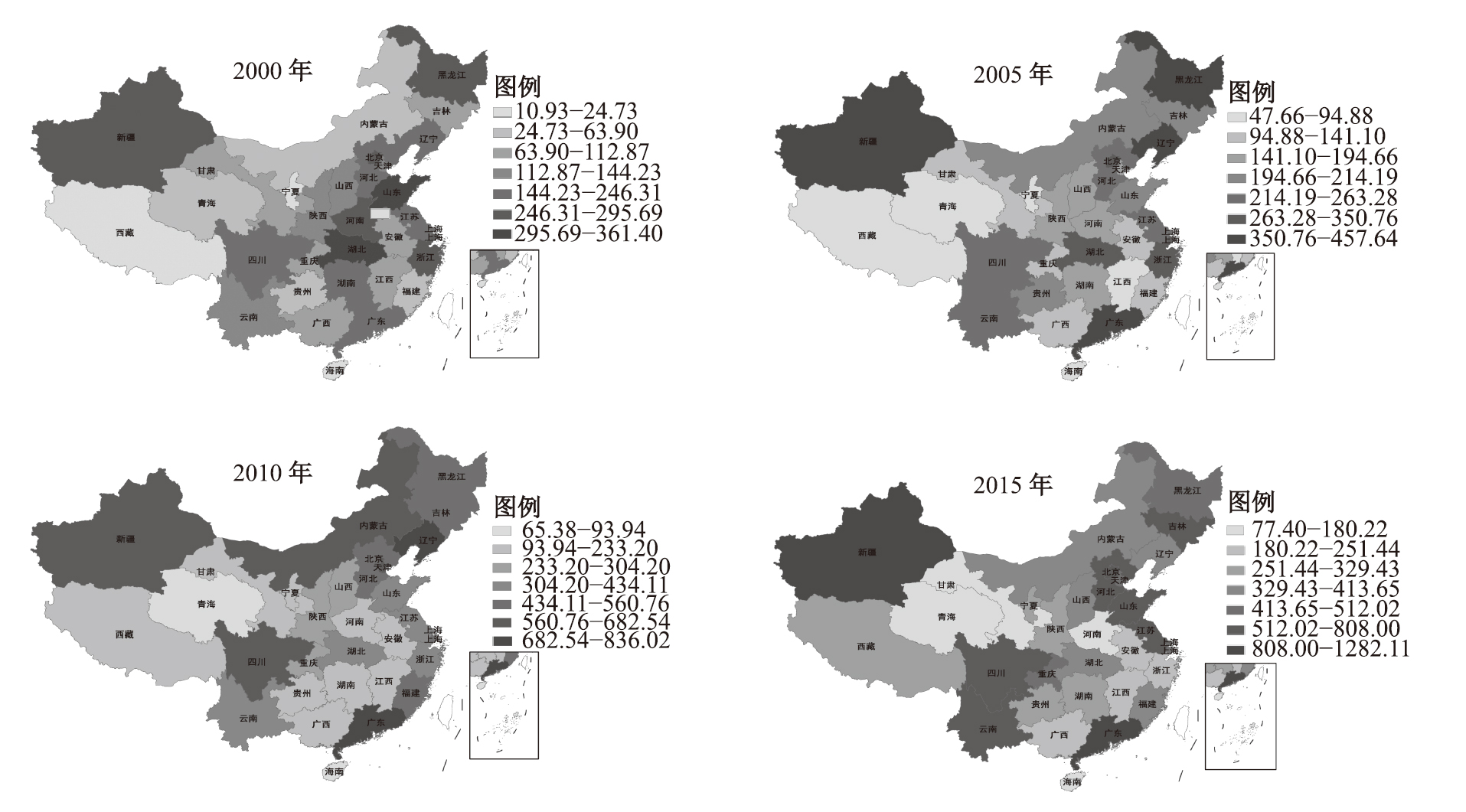﻿ 中央投资项目跨区配置的决定因素：经济准则还是政治影响力
 上海财经大学学报2018, Vol. 20Issue (5): 30-430

#### 文章信息上海财经大学2018年20卷第5期

Yuan Deyu, Song Xiaoning, Li Degang

Determinants of Central Investment Across Chinese Regions: Economic Normative Criteria or Political Clout

Journal of Shanghai University of Finance and Economics, 2018, 20(5): 30-43.

### 文章历史《上海财经大学学报》
2018第20卷第5期

1.对外经济贸易大学　国际经济贸易学院，北京　100029;
2.中山大学管理学院/现代会计与财务研究中心，广东　广州　510275;
3.北京第二外国语学院　经贸与会展学院，北京　100024

(一) 代表性地区的生产函数

 ${Y_{it}} = {A_i}K_{it}^\alpha L_{it}^{1 - \alpha }S_{it}^\gamma \quad\alpha ,\gamma \in \left( {0,1} \right)$ (1)

 ${S_{it}} = {{{G_{it}}}/{{\xi _i}}}$ (2)

 ${G_{it}} = I_{it}^C + (1 - \delta ){G_{it - 1}}$ (3)
(二) 中央投资决策

 ${{\rm{W}}_t} = {\sum\nolimits_i {{L_{it}}\left( {{Y_{it}}/{L_{it}}} \right)} ^\varphi }{\rm{ + }}1/\eta \ln \left[ {\sum\nolimits_i {{L_{it}}\Theta _{it}^{}{{\left( {{Y_{it}}/{L_{it}}} \right)}^\eta }} } \right]$ (4)

 $\sum\nolimits_i {I_{it}^C} \leqslant \sum\nolimits_i {\tau {Y_{it}}}$ (5)

 $LAG = {\sum\nolimits_i {{L_{it}}\left( {{Y_{it}}/{L_{it}}} \right)} ^\varphi }{\rm{ + }}(1/\eta )\ln \left[ {\sum\nolimits_i {{L_{it}}\Theta _{it}^{}{{\left( {{Y_{it}}/{L_{it}}} \right)}^\eta }} } \right] - {\lambda _t}(\sum\nolimits_i {I_{it}^C} - \sum\nolimits_i {\tau {Y_{it}}} )$ (6)

 $\left[ {\varphi \times {{\left( {\frac{{{Y_{it}}}}{{{L_{{\rm{it}}}}}}} \right)}^{\varphi - 1}}{\rm{ + }}\frac{{\Theta _{it}^{}}}{{{e^{\eta {U_t}}}}} \times {{\left( {\frac{{{Y_{it}}}}{{{L_{it}}}}} \right)}^{\eta - 1}}{\rm{ + }}\tau {\lambda _{\rm{t}}}} \right] \times \frac{{{Y_{it}}}}{{{G_{it}}}} \times \frac{{\partial {G_{it}}}}{{\partial I_{it}^C}} = \frac{{{\lambda _t}}}{\gamma }$ (7)

 $G_{it}^* = \frac{\gamma }{{{\lambda _t}}} \times \left[ {\varphi \times {{\left( {\frac{{{Y_{it}}}}{{{L_{it}}}}} \right)}^{\varphi - 1}} + \frac{{\Theta _{it}^{}}}{{{e^{\eta {U_t}}}}} \times {{\left( {\frac{{{Y_{it}}}}{{{L_{it}}}}} \right)}^{\eta - 1}} + \tau {\lambda _t}} \right] \times {Y_{it}}$ (8)

 ${G_{it}} - {G_{it - 1}} = \pi (G_{it - 1}^* - {G_{it - 1}})$ (9)

 $\frac{{I_{it}^C}}{{{G_{it - 1}}}} = \frac{{\pi \gamma }}{{{\lambda _{t - 1}}}} \times \left[ {\varphi \times {{\left( {\frac{{{Y_{it - 1}}}}{{{L_{it - 1}}}}} \right)}^{\varphi {\rm{ - }}1}} + \frac{{\Theta _{it - 1}^{}}}{{{e^{\eta {U_{t - 1}}}}}} \times {{\left( {\frac{{{Y_{it - 1}}}}{{{L_{it - 1}}}}} \right)}^{\eta {\rm{ - }}1}} + \tau {\lambda _{t - 1}}} \right] \times \frac{{{Y_{it - 1}}}}{{{G_{it - 1}}}} + (\delta - \pi )$ (10)

 ${{\partial \frac{{I_{it}^C}}{{{G_{it - 1}}}}}/\partial }\frac{{{Y_{it - 1}}}}{{{L_{it - 1}}}} = \frac{{\pi \gamma }}{{{\lambda _{t - 1}}}} \times \left[ {\varphi (\varphi - 1) \times {{\left( {\frac{{{Y_{it - 1}}}}{{{L_{it - 1}}}}} \right)}^{\varphi {\rm{ - 2}}}} + (\eta - 1) \times \frac{{\Theta _{it - 1}^{}}}{{{e^{\eta {U_{t - 1}}}}}} \times {{\left( {\frac{{{Y_{it - 1}}}}{{{L_{it - 1}}}}} \right)}^{\eta {\rm{ - 2}}}}} \right] \times \frac{{{Y_{it - 1}}}}{{{G_{it - 1}}}} < 0$ (11)
 ${{\partial \frac{{I_{it}^C}}{{{G_{it - 1}}}}}/\partial }\frac{{{Y_{it - 1}}}}{{{G_{it - 1}}}} = \frac{{\pi \gamma }}{{{\lambda _{t - 1}}}} \times \left[ {\varphi \times {{\left( {\frac{{{Y_{it - 1}}}}{{{L_{it - 1}}}}} \right)}^{\varphi {\rm{ - }}1}} + \frac{{\Theta _{it - 1}^{}}}{{{e^{\eta {U_{t - 1}}}}}} \times {{\left( {\frac{{{Y_{it - 1}}}}{{{L_{it - 1}}}}} \right)}^{\eta {\rm{ - }}1}} + \tau {\lambda _{t - 1}}} \right] > 0$ (12)
 ${{\partial \frac{{I_{it}^C}}{{{G_{it - 1}}}}}/\partial }{\Theta _{it - 1}} = \frac{{\pi \gamma }}{{{\lambda _{t - 1}}{e^{\eta {U_{t - 1}}}}}} \times {\left( {\frac{{{Y_{it - 1}}}}{{{L_{it - 1}}}}} \right)^{\eta {\rm{ - }}1}} \times \frac{{{Y_{it - 1}}}}{{{G_{it - 1}}}} > 0$ (13)

 \begin{aligned} {{I_{it}^C}/{{G_{it - 1}}}} = &{\beta _0} + {\beta _1} \times \ln ({Y_{it - 1}}/{G_{it - 1}}) + {\beta _2} \times \ln ({Y_{it - 1}}/{L_{it - 1}}) + {\beta _3} \times {\Theta _{it - 1}} \\ &{\rm{ }} + {{ Z}^{\bf{'}}}{ X} + {{ H}^{\bf{'}}}{ D} + {\mu _i} + {\varepsilon _{it}} \\ \end{aligned} (14)

 \begin{aligned} {{I_{it}^C}/{{G_{it - 1}}}} =& {\beta _0} + \beta \sum\nolimits_j {{{{w_{ij}}(I_{jt}^C}/{{G_{jt - 1}})}}} + {\beta _1} \times \ln ({Y_{it - 1}}/{G_{it - 1}}) + {\beta _2} \times \ln ({Y_{it - 1}}/{L_{it - 1}}) + {\beta _3} \times {\Theta _{it - 1}} \\ &{\rm{ }} + {{Z}}^{\bf{'}}{{X}} + {{H}^{\bf{'}}}{{D}}+ {\mu _i} + {\varepsilon _{it}} \\ \end{aligned} (15)

(二) 估计方法

(三) 变量与数据

（1）中央投资（ ${{I_{it}^C}/{{G_{it - 1}}}}$ ）。这个指标数据的获取，涉及中央对地方的政府投资额和地方基础设施水平的确定。在国内，目前并没有文献专门针对“政府投资”进行统计。借鉴王曦等（2014）的做法，本文将《中国统计年鉴》中统计列示的“中央项目”近似衡量“中央投资”。图1展示了2000年、2005年、2010年和2015年中央投资项目的空间分布情况。对于“基础设施水平”，目前有两类通行的衡量方法：一是用资本存量度量；二是用实物存量度量。尽管当前已有很多文献对中国省级地方公共资本存量做过测算（金戈，2012），但终因这些测算是基于诸多理论假设进行的，所得结果的准确性自然也就有所欠缺。在本文中，我们舍弃已有的测算公共资本存量的方法和数据，尝试通过基础设施实物存量构建一个省级“基础设施综合指数”来对基础设施水平进行度量。图 1 中央投资项目的空间分布情况 数据来源：相关年份的《中国统计年鉴》。图 2 1998–2015年省级基础设施水平综合指数的变化 数据来源：各年份《中国统计年鉴》以及作者计算。

（2）单位基础设施产出水平（Yit/Git）和人均产出水平（Yit/Lit）。与上文理论模型中设定涵义相一致，这两个指标分别用于描述中央投资对于效率因素和公平（再分配）因素的考虑。其中，Y用“省级地区GDP水平”进行衡量；L用“省级地区的年初和年末常住人口数的算术平均值”来衡量。

（3）政治影响力。此指标被界定为一个地区对中央的影响力或者在中央决策层的代表性（李明和李慧中，2010），我们通过构建“政治影响力”指标对其进行衡量。在中国的政治体制下，考虑到中共中央委员会在国家经济和社会资源配置中的重要作用，我们选用各省份拥有的中央委员（包括政治局委员和中央候补委员）来构建地方的“政治影响力”指标。参照吴凤武等（2013）的做法，我们计算某年在某地任职的地方官员同时位居中央政治局委员、中央委员、中央候补委员的人数，按每人10分、2分和1分的标准分别赋予任职地相应的政治影响力得分，并逐年加总各省（自治区或直辖市）的得分，得到来自地方的政治影响力指标，即“地方政治影响力”（local）。另外，考虑到一个地区的政治影响力还可能来源于与这个地区有一定关联但目前在中央任职的官员，因此，我们还按照“籍贯地”（或“出生地”）和“任现职前主要任职地”两个标准分别对在中央任职的中央委员按省份进行人数归并，同样按照“地方政治影响力”指标对中央政治局委员、中央委员和中央候补委员的赋分标准，构建两个来自中央的政治影响力指标，即“籍贯地政治影响力”（jgd）和“任职地政治影响力”（rzd）。此外，考虑到国家发展与改革委员会对中央投资具有重要的配置权力，我们还将此部门当年在任的主任和副主任分别参照中央委员和中央候补委员的标准赋分，计入上述两个中央政治影响力指标的分值中。需要指出的是，我们在统计中央委员人数时，并未把军队系统考虑在内，这是因为军队系统通常是独立封闭运行的，与地方的经济社会发展并无太多直接利益关系。

 变量 样本数 均值 标准差 最小值 最大值 中央投资（ ${{{I_{it}^C}/{{G_{it - 1}}}}}$ ） 496 2.618 1.690 0.255 12.856 单位基础设施产出水平（ ${{Y_{it}}/{G_{it}}}$ ） 496 58.224 32.454 2.651 184.698 人均产出水平（ ${{Y_{it}}/{L_{it}}}$ ） 496 2.012 1.463 0.271 8.232 地方政治影响力（ ${loca{l_{it}}}$ ） 496 7.397 3.964 2.000 20.000 地方社会投资水平（ ${loci{n_{it}}}$ ） 496 13.554 8.832 0.208 57.553 城镇化水平（ ${ur{b_{it}}}$ ） 496 46.382 14.785 18.204 89.600 人口密度（ ${popde{n_{it}}}$ ） 496 4.087 5.869 0.021 3 38.384 工业化水平（ ${indu{s_{it}}}$ ） 496 39.682 9.927 7.026 57.319 财政分权（ ${fc{e_{it}}}$ ） 496 77.600 11.033 31.831 95.671

 静态模型 动态模型 （ⅰ）MLE （ⅱ）MLE （ⅲ）SYS-GMM （ⅳ）SYS-GMM 因变量一阶滞后项 0.718***（7.17） 0.811***（6.07） ${\ln ({Y_{it - 1}}/{G_{it - 1}})}$ 2.670***（21.00） 2.452***（18.19） 0.203**（2.06） 0.220*（1.67） ${\ln ({Y_{it - 1}}/{G_{it - 1}})}$ –0.229（–0.73） –0.016 4（–0.13） 0.531（0.50） 0.490（1.35） ${loca{l_{it - 1}}}$ 0.089 4***（4.46） 0.060 6***（3.21） 0.045 0***（3.22） 0.018 3**（2.16） ${W.(I_{it}^C/{G_{it - 1}})}$ 0.003 58（0.58） 0.011 2*（1.73） 0.061 0***（3.99） 0.096 8***（2.87） ${loci{n_{it}}}$ 0.007 40（0.88） –0.036 4*（–1.95） ${ur{b_{it}}}$ 0.003 10（0.59） 0.016 1（1.16） ${indu{s_{it}}}$ –0.006 24（–1.10） 0.016 8**（2.53） ${popde{n_{it}}}$ –0.011 5（–1.19） 0.013 8（0.86） ${fc{e_{it}}}$ 0.005 56*（1.68） –0.013 9*（–1.69） ${{R^2}}$ 0.534 0.630 0.775 0.847 LogL –659.7 –677.5 –448.97 –489.3 Robust LM_LAG 3. 105** 4. 050** 40.42*** 13.87*** Robust LM_ERROR 0.170 0.294 17.591*** 0.137 观察值数 496 496 465 465 注：（1）***、**和*分别表示1％、5％和10％的显著性水平。（2）圆括号中数据为异方差稳健的t统计量，表3和表4同。（3）Robust LM_LAG是空间滞后效应拉格朗日乘子稳健统计量（服从卡方分布），而Robust LM_ERROR是空间误差效应拉格朗日乘子稳健统计量（服从卡方分布）。这两个指标用于检验实证模型中加入空间变量的必要性和合理性，其检验的原假设为：空间滞后因变量或误差项不存在空间自相关。

(二) 分时段考察

 1998–2007年 2008–2015年 SYS（ⅰ） SYS（ⅱ） SYS（ⅲ） SYS（ⅳ） 因变量一阶滞后项 0.943***（10.32） 0.917***（6.61） 0.618***（5.83） 0.650***（3.18） ${\ln ({Y_{it - 1}}/{G_{it - 1}})}$ –0.709（–0.80） 0.184（0.55） 0.267**（2.42） 0.429**（2.00） ${\ln ({Y_{it - 1}}/{L_{it - 1}})}$ 0.632***（4.08） 0.462**（2.33） 0.640**（2.04） –0.029 3（–1.05） ${loca{l_{it - 1}}}$ 0.012 6***（2.77） 0.024 3**（2.27） 0.050 3（1.54） 0.060 0（1.34） ${W.(I_{it}^C/{G_{it - 1}})}$ 0.037 5***（2.82） 0.035 2***（2.95） 0.035 6**（2.18） 0.022 4*（1.80） 控制变量 NO YES NO YES ${{R^2}}$ 0.892 0.930 0.745 0.876 LogL –83.6 –74.0 –235.5 –231.2 观察值数 217 217 217 217

 使用中央政治影响力变量 改变地方政治影响力赋值 扩展空间权重矩阵 ${jgd}$ ${rzd}$ local1 local2 ${W2}$ ${W3}$ SYS（ⅰ） SYS（ⅱ） SYS（ⅲ） SYS（ⅳ） SYS（ⅴ） SYS（ⅵ） 因变量一阶滞后项 0.799***（9.87） 0.817***（10.72） 0.785***（10.26） 0.782***（10.24） 0.836***（5.73） 0.797***（5.71） ${\ln ({Y_{it - 1}}/{G_{it - 1}})}$ 0.401***（3.24） 0.354***（2.87） 0.419***（3.46） 0.437***（3.63） 0.256*（1.72） 0.157（1.49） ${\ln ({Y_{it - 1}}/{L_{it - 1}})}$ 0.250（1.52） 0.212（1.16） 0.199（1.02） 0.216（1.22） 0.322（1.55） 0.276（1.07） ${loca{l_{it - 1}}}$ 或 ${loc{a_{it - 1}}}$ 或 ${loc{b_{it - 1}}}$ 0.017 7**（2.40） 0.016 8**（1.98） 0.022 6***（3.03） 0.030 1***（2.76） ${jg{d_{it - 1}}}$ 或 ${rz{d_{it - 1}}}$ –0.004 23（–0.60） 0.006 33**（2.04） ${W.(I_{it}^C/{G_{it - 1}})}$ 0.020 1**（2.21） 0.017 3*（1.82） 0.013 3**（2.41） 0.018 3*（1.94） ${W1.(I_{it}^C\!/\!{G_{it - 1}})}$ 或 ${W2.(I_{it}^C\!/\!{G_{it - 1}})}$ 12.117*（1.76） 0.068 6***（3.42） 控制变量 YES YES YES YES YES YES ${{R^2}}$ 0.802 0.799 0.800 0.796 0.859 0.868 LogL –441.8 –441.0 –439.3 –440.2 –469.8 –473.7 观察值数 465 465 465 465 465 465

① 在中国，地方政府为中央社会经济政策的执行机构，相应地，“中央政府”实质性地成为了“国家政府”。因此，中央政府在安排投资时通常会从国家整体考虑，其不仅要从中央层面去关注投资对其本身产生的福利，而且还要关注国家投资项目落地后为其辖区带来的社会福利情况。

② 也有文献使用Y/GY/L两个指标衡量影响政府投资的效率因素和公平因素，如Kemmerling等（2015）。我们知道，政府投资通常形成基础设施，在其他条件一定的情况下，单位基础设施所带来的产出越多，表示之前的政府投资就越有效率；政府投资可以直接或间接促进经济发展，如果一个相对较发达的地区，中央政府仍加强对其进行投资而减少对欠发达地区投资，那么无疑会加剧地区之间的经济发展不平衡或不公平程度。

③ 海南省与其他省份并无陆域邻接，但考虑到该省地理位置及与其他地区的经济往来情况，本文设定其与广东省、广西壮族自治区具有共同边界。

④ 政府投资项目特别是中央项目的周期通常超过1年，并且当前我国政府预算的编制采用的方法仍是基数法，当年预算是以上年度的预决算为基础进行预测、调整而编制完成的，因此假定中央投资跨期性有理论上的合理性。

⑤ 国内文献对“政府投资”数据的处理主要采用加总法，即加总财政支出中 “基本建设费”“企业挖潜改造”“科技三项费用”“城市维护建设费”“支援不发达地区建设费”等具有投资性支出项目的决算额，如张卫国等（2010）、苑德宇（2014）等。这种方法虽然有针对性地反映了中国政府投资性支出情况，但是因为1998年后至今官方财政支出统计口径和国民经济行业口径均发生了变化，这严重地影响了利用上述方法获得“政府投资”数据的连贯性甚至可得性。本文之所以借鉴王曦等（2014）的做法，利用固定资产投资中“中央项目”（主要包括中央预算内投资资金、专项建设基金、国家主权外债资金等中央财政投资性资金项目）表示“中央对地方的政府投资”主要出于数据可得性考虑，尽管用于“中央项目”的固定资产投资资金并不完全来源于公共部门，但因项目由政府主导，所建设施通常具有公共性，我们把其中使用的民间资金也视为政府投资，具有一定的合理性。

⑥ 在处理“政治影响力”指标时，如果遇到中央委员的“籍贯地”和“出生地”不同时，我们综合考虑该官员背景，将其适当地归入“籍贯地”或“出生地”。“任现职前主要任职地”的界定标准为：如果官员擢升为现职前最近地方任职的年限超过1年（包括1年），我们以该最近任职所在地作为其“任职地”；如果上述任职年限低于1年，我们则以该官员最长地方任职地作为“任职地”。当然如果一个官员没有地方任职经历，我们不对其进行“任职地”归并处理，仅将其规定到“籍贯地”（“出生地”）中。

⑦ 本文按照少数民族人口数占全省人口数的比例超过15%的标准划定某一省份是否为少数民族地区。本文利用1998–2015年全国各省的人口数据进行综合计算，选定内蒙古、辽宁、广西、海南、贵州、云南、西藏、青海、宁夏和新疆10省份为少数民族地区。

⑧ 设定矩阵元素wij为两省份距离（省会城市之间公路里程）的倒数。

⑨ 我们将经济发展水平纳入影响相邻地区间中央投资空间联系的因素中，构建了此空间权重矩阵。具体设定为： ${W2 = W \times diag({\bar y_1}/\bar y,{\bar y_2}/\bar y, \cdot \cdot \cdot ,{\bar y_n}/\bar y)}$ ，其中， ${\bar y_i}$ 为样本期内第i个省份实际人均产出水平的平均值， ${\bar y}$ 为样本期内实际人均产出水平的总平均值。

  何庆光. 财政分权对地方公共投资的长期和短期影响效应研究——基于省级面板数据的实证分析[J].经济理论与经济管理,2010(7).  金戈. 中国基础设施资本存量估算[J].经济研究,2012(4).  李明, 李慧中. 政治资本与中国的地区收入差异[J].世界经济文汇,2010(5).  王曦, 杨扬, 余壮雄, 等. 中央投资对中国区域资本流动的影响[J].中国工业经济,2014(4).  吴凤武, 刘琦, 胡祖铨. 中央对地方转移支付影响因素分析——地方政府政治力量的作用研究[J].经济研究参考,2013(67).  苑德宇. 地方政府投资的决定因素研究: 基于税收预决算偏离的视角[J].世界经济,2014(8).  张军, 高远, 傅勇, 等. 中国为什么拥有了良好的基础设施?[J].经济研究,2007(3).  张卫国, 任燕燕, 花小安. 地方政府投资行为、地区性行政垄断与经济增长——基于转型期中国省级面板数据的分析[J].经济研究,2011(8).  Alonso-Carrera J, Freire-Serén M J, Manzano B. Macroeconomic effects of the regional allocation of public capital formation[J]. Regional Science and Urban Economics,2009,139(5):563–574.  Anselin L, Moreno R. Properties of tests for spatial error components[J].Regional Science and Urban Economics,2003,33(5):595–618.  Arulampalam W, Dasgupta S, Dhillon A, et al. Electoral goals and center-state transfers: A theoretical model and empirical evidence from India[J].Journal of Development Economics,2009,88(1):103–119.  Barro R J. Government spending in a simple model of endogeneous growth[J]. Journal of Political Economy,1990,198(5):103–125.  Behrman J, Craig S. The distribution of public services: An exploration of local governmental preferences[J]. American Economic Review,1987,177(1):37–49.  Blundell R, Bond S. Initial conditions and moment restrictions in dynamic panel data models[J].Journal of Econometrics,1998,87(1):115–143.  Boyle M A, Matheson V A. Determinants of the distribution of congressional earmarks across states[J].Economics Letters,2009,104(2):63–65.  Cadot O, Röller L H, Stephan A. Contribution to productivity or pork barrel? The two faces of infrastructure investment[J].Journal of Public Economics,2006,90(6-7):1133–1153.  Castells A, Solé-Ollé A. The regional allocation of infrastructure investment: The role of equity, efficiency and political factors[J].European Economic Review,2005,49(5):1165–1205.  Duchin R, Sosyura D. The politics of government investment[J].Journal of Financial Economics,2012,106(1):24–48.  Kemmerling A, Stephan A. Comparative political economy of regional transport infrastructure investment in Europe[J]. Journal of Comparative Economics,2015,143(1):227–239.  Lambrinidis M, Psycharis Y, Rovolis A. Regional allocation of public infrastructure investment: The case of Greece[J].Regional Studies,2005,39(9):1231–1244.  Oates W E. An essay on fiscal federalism[J].Journal of Economic Literature,1999,37(3):1120–1149.  Sturm J. Determinants of public capital spending in less-developed countries[R]. University of Groningen &CESifo Munich Working Paper, 2001.  Wallis J J. The political economy of new deal spending revisited, again: With and without Nevada[J].Explorations in Economic History,1998,35(2):140–170.  Worthington A C, Dollery B E. The political determination of intergovernmental grants in Australia[J]. Public Choice,1998,94(3-4):299–315.  Yang D L L. Economic transformation and its political discontents in China: Authoritarianism, unequal growth, and the dilemmas of political development[J].Annual Review of Political Science,2006,9:143–164.  Zheng X Y, Li H, Song F, et al. Central government’s infrastructure investment across Chinese regions: A dynamic spatial panel data approach[J].China Economic Review,2013,27:264–276.
Determinants of Central Investment Across Chinese Regions: Economic Normative Criteria or Political Clout
Yuan Deyu1, Song Xiaoning2, Li Degang3
1.University of International Business and Economics，Beijing 100029，China;
2.Sun Yet-sen University，Guangdong Guangzhou 510275，China;
3.Beijing International Studies University，Beijing 100024，China
Summary: The central government investment usually guides the local investment, and accomplishes a great task with little effort by clever maneuvers in the rapid economy growth in the past thirty years. Faced with the widening gap in regional development, the distribution of central investment projects should pay more attention to equity or efficiency factors? Answering this question is helpful to explain the disparity and change of the regional development level from the perspective of central investment. In addition, the provincial political influence may also " interfere” with the efficiency and fairness of central investment projects. 　　This paper extends the theoretical model of central investment decision-making, and puts forward the theoretical hypothesis of central investment on economic criteria and political influences. The " political influence” factor is measured by the number of Central Committee members (including Politburo members and central alternate members) in each province. The supreme organ of power is the Central Committee of the Communist Party of China. The most important decisions are usually made by the Central Committee. The number of Central Committee members in each province determines the political influence of each province. We use the unit infrastructure output level and per capita GDP to measure efficiency factors and equity factors respectively. 　　This paper constructs a spatial dynamic panel model and conducts an empirical test by using Chinese 1998-2015 inter-provincial data. The empirical results show that both efficiency and the political influence have a significant positive impact on central investment, while equity is insignificant. In cross-temporal comparison, the impact of efficiency factors on central investment is insignificant in 1998-2007 and positive in 2008-2015; while the impact of equity and the political influence changes from significant positive to insignificant.　　The policy implication of these empirical findings is that policy adjustments should be made in the following two aspects in order that central investment projects play a more effective role in promoting growth, adjusting the structure and benefiting people’s livelihood. On the one hand, reduce political interference, and strengthen budget management and approval of central investment funds. On the other hand, adjust and optimize the scale and structure of central investment, put more central projects into public services, and coordinate regional economic development, which will more effectively promote the transformation of central investment from the single objective of efficiency to the goal of both equity and efficiency.
Key words: central investment     economic normative criteria     political clout     spatial dynamic panel model